# 图形界面 X: 在多行文本框中选择文本 (集成构建 13)

### 内容目录

• 概述
• 跟踪 Shift 键的按压
• 选择文本的组合键
• 选择文本的方法
• 删除已选文本的方法
• 操纵图像数据的类
• 结论

### 概述

• 图形界面 X: 多行文本框控件 (集成构建 8)
• 图形界面 X: 在多行文本框中回卷文本的算法 (集成构建 12)

### 跟踪 Shift 键的按压

```//+------------------------------------------------------------------+
//| 操控键盘的类                                                       |
//+------------------------------------------------------------------+
class CKeys
{
public:
//--- 返回 Shift 键的状态
bool              KeyShiftState(void);
};
//+------------------------------------------------------------------+
//| 返回 Shift 键的状态                                                |
//+------------------------------------------------------------------+
bool CKeys::KeyShiftState(void)
{
return(::TerminalInfoInteger(TERMINAL_KEYSTATE_SHIFT)<0);
}

```

### 选择文本的组合键

• 'Shift + Left' 和 'Shift + Right' 组合可将文本光标分别向左或向右移动一个字符。文本以不同的背景和字符颜色高亮显示 (也可由用户自行定义):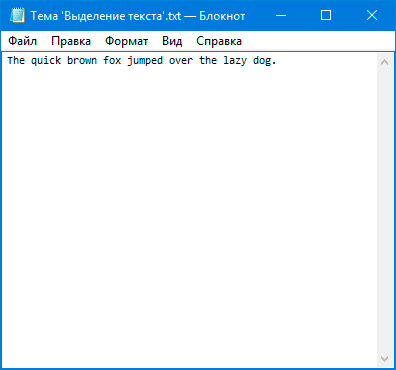• 'Shift + Home' 和 'Shift + End' 组合可将文本光标移至行首或行尾, 并从光标的起始位置选择所有字符。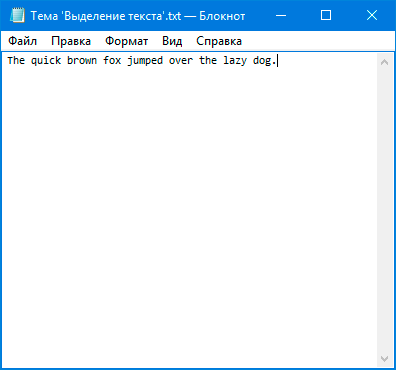• 'Shift + Up' 和 'Shift + Down' 组合可将文本光标分别向上或向下移一行。选择文本则自光标处到起始行的开头, 或自最后一行的行尾到光标处。如果在初始和最终选择的行之间有更多行, 则其内文本将被完全选择。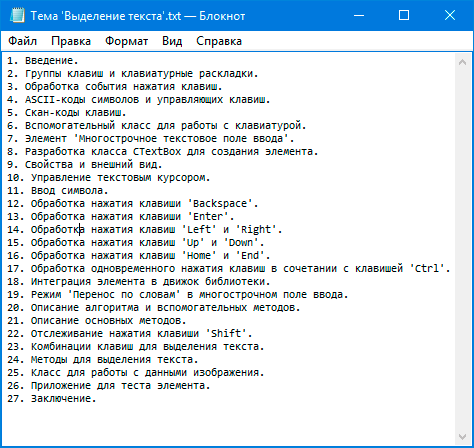• 'Ctrl + Shift + Left' 和 'Ctrl + Shift + Right' 组合分别用于在文本光标当前位置的左侧和右侧选择整个单词: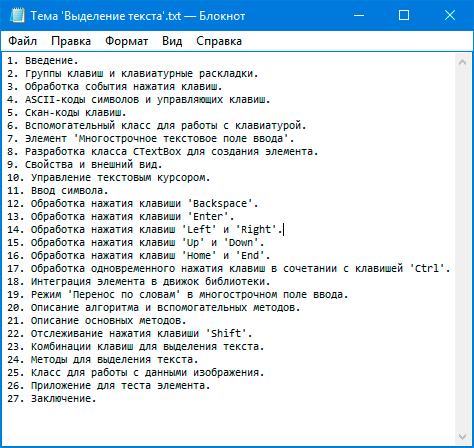• 'Ctrl + Shift + Home' 和 'Ctrl + Shift + End' 组合可以选择自文本光标的当前位置开始至首行开头或最后一行的末尾间的所有文本: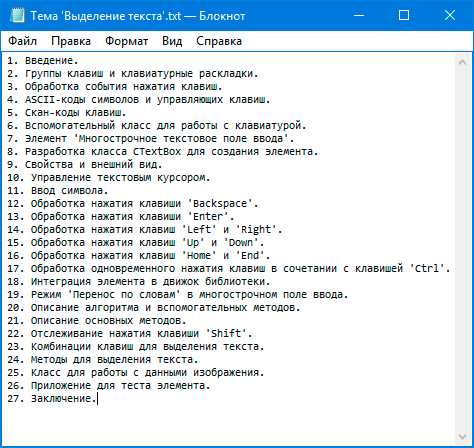### 选择文本的方法

```class CTextBox : public CElement
{
private:
//--- 所选文本的背景和字符颜色
color             m_selected_back_color;
color             m_selected_text_color;
//---
private:
//--- 所选文本的背景和字符颜色
void              SelectedBackColor(const color clr)        { m_selected_back_color=clr;       }
void              SelectedTextColor(const color clr)        { m_selected_text_color=clr;       }
};
//+------------------------------------------------------------------+
//| 构造器                                                            |
//+------------------------------------------------------------------+
CTextBox::CTextBox(void) : m_selected_text_color(clrWhite),
m_selected_back_color(C'51,153,255')
{
//...
}

```

```class CTextBox : public CElement
{
private:
//--- 行和字符 (所选文本的) 的起始行和结束行索引
int               m_selected_line_from;
int               m_selected_line_to;
int               m_selected_symbol_from;
int               m_selected_symbol_to;
//---
private:
//--- 设置所选文本的 (1) 起始行 (2) 结束行索引
void              SetStartSelectedTextIndexes(void);
void              SetEndSelectedTextIndexes(void);
//--- 重置所选文本
void              ResetSelectedText(void);
};
//+------------------------------------------------------------------+
//| 设置所选文本的起始行索引                                             |
//+------------------------------------------------------------------+
void CTextBox::SetStartSelectedTextIndexes(void)
{
//--- 如果尚未设置选择文本的起始行索引
if(m_selected_line_from==WRONG_VALUE)
{
m_selected_line_from   =(int)m_text_cursor_y_pos;
m_selected_symbol_from =(int)m_text_cursor_x_pos;
}
}
//+------------------------------------------------------------------+
//| 设置所选文本的结束行索引                                              |
//+------------------------------------------------------------------+
void CTextBox::SetEndSelectedTextIndexes(void)
{
//--- 设置所选文本的结束行索引
m_selected_line_to   =(int)m_text_cursor_y_pos;
m_selected_symbol_to =(int)m_text_cursor_x_pos;
//--- 如果所有索引相同, 则清除选择
if(m_selected_line_from==m_selected_line_to && m_selected_symbol_from==m_selected_symbol_to)
ResetSelectedText();
}
//+------------------------------------------------------------------+
//| 重置所选文本                                                       |
//+------------------------------------------------------------------+
void CTextBox::ResetSelectedText(void)
{
m_selected_line_from   =WRONG_VALUE;
m_selected_line_to     =WRONG_VALUE;
m_selected_symbol_from =WRONG_VALUE;
m_selected_symbol_to   =WRONG_VALUE;
}

```

```class CTextBox : public CElement
{
private:
//--- 将文本光标向左移动一个字符
void              MoveTextCursorToLeft(void);
//--- 将文本光标向右移动一个字符
void              MoveTextCursorToRight(void);
//--- 将文本光标向上移动一个字符
void              MoveTextCursorToUp(void);
//--- 将文本光标向下移动一个字符
void              MoveTextCursorToDown(void);

//--- 调整水平滚动条
void              CorrectingHorizontalScrollThumb(void);
//--- 调整垂直滚动条
void              CorrectingVerticalScrollThumb(void);
};

```

• TO_NEXT_LEFT_SYMBOL — 向左一个字符。
• TO_NEXT_RIGHT_SYMBOL — 向右一个字符。
• TO_NEXT_LEFT_WORD — 向左一个单词。
• TO_NEXT_RIGHT_WORD — 向右一个单词。
• TO_NEXT_UP_LINE — 向上一行。
• TO_NEXT_DOWN_LINE — 向下一行。
• TO_BEGIN_LINE — 至当前行的开头。
• TO_END_LINE — 至当前行的末尾。
• TO_BEGIN_FIRST_LINE — 至当首行的开头。
• TO_END_LAST_LINE — 至最后一行的末尾。
```//+------------------------------------------------------------------+
//| 移动文本光标的方向枚举                                               |
//+------------------------------------------------------------------+
enum ENUM_MOVE_TEXT_CURSOR
{
TO_NEXT_LEFT_SYMBOL  =0,
TO_NEXT_RIGHT_SYMBOL =1,
TO_NEXT_LEFT_WORD    =2,
TO_NEXT_RIGHT_WORD   =3,
TO_NEXT_UP_LINE      =4,
TO_NEXT_DOWN_LINE    =5,
TO_BEGIN_LINE        =6,
TO_END_LINE          =7,
TO_BEGIN_FIRST_LINE  =8,
TO_END_LAST_LINE     =9
};

```

```class CTextBox : public CElement
{
private:
//--- 按指定的方向移动文本光标
void              MoveTextCursor(const ENUM_MOVE_TEXT_CURSOR direction);
};
//+------------------------------------------------------------------+
//| 按指定的方向移动文本光标                                             |
//+------------------------------------------------------------------+
void CTextBox::MoveTextCursor(const ENUM_MOVE_TEXT_CURSOR direction)
{
switch(direction)
{
//--- 光标向左移动一个字符
case TO_NEXT_LEFT_SYMBOL  : MoveTextCursorToLeft();        break;
//--- 光标向右移动一个字符
case TO_NEXT_RIGHT_SYMBOL : MoveTextCursorToRight();       break;
//--- 光标向左移动一个单词
case TO_NEXT_LEFT_WORD    : MoveTextCursorToLeft(true);    break;
//--- 光标向右移动一个单词
case TO_NEXT_RIGHT_WORD   : MoveTextCursorToRight(true);   break;
//--- 光标向上移动一行
case TO_NEXT_UP_LINE      : MoveTextCursorToUp();          break;
//--- 光标向下移动一行
case TO_NEXT_DOWN_LINE    : MoveTextCursorToDown();        break;
//--- 光标移动到当前行的开头
case TO_BEGIN_LINE : SetTextCursor(0,m_text_cursor_y_pos); break;
//--- 光标移动到当前行的末尾
case TO_END_LINE :
{
//--- 获取当前行中的字符数
uint symbols_total=::ArraySize(m_lines[m_text_cursor_y_pos].m_symbol);
//--- 移动光标
SetTextCursor(symbols_total,m_text_cursor_y_pos);
break;
}
//--- 光标移动到首行的开头
case TO_BEGIN_FIRST_LINE : SetTextCursor(0,0); break;
//--- 光标移动到最后一行的末尾
case TO_END_LAST_LINE :
{
//--- 获取最后一行的行号和字符数
uint lines_total   =::ArraySize(m_lines);
uint symbols_total =::ArraySize(m_lines[lines_total-1].m_symbol);
//--- 移动光标
SetTextCursor(symbols_total,lines_total-1);
break;
}
}
}

```

```//+------------------------------------------------------------------+
//| 处理 Left 键按压                                                   |
//+------------------------------------------------------------------+
bool CTextBox::OnPressedKeyLeft(const long key_code)
{
//--- 如果 (1) 并非 Left 键, 或是 (2) 按下 Ctrl 键, 或是 (3) 按下 Shift 键, 离开
if(key_code!=KEY_LEFT || m_keys.KeyCtrlState() || m_keys.KeyShiftState())
return(false);
//--- 重置选择
ResetSelectedText();
//--- 文本光标向左移动一个字符
MoveTextCursor(TO_NEXT_LEFT_SYMBOL);
//--- 调整滚动条
CorrectingHorizontalScrollThumb();
CorrectingVerticalScrollThumb();
//--- 更新文本框中的文本
DrawTextAndCursor(true);
//--- 发送有关它的消息
::EventChartCustom(m_chart_id,ON_MOVE_TEXT_CURSOR,CElementBase::Id(),CElementBase::Index(),TextCursorInfo());
return(true);
}

```

```//+------------------------------------------------------------------+
//| 处理按下 Shift + Left 键                                           |
//+------------------------------------------------------------------+
bool CTextBox::OnPressedKeyShiftAndLeft(const long key_code)
{
//--- 如果 (1) 并非 Left 键, 或是 (2) 按下 Ctrl 键, 或是 (3) 未按下 Shift 键, 离开
if(key_code!=KEY_LEFT || m_keys.KeyCtrlState() || !m_keys.KeyShiftState())
return(false);
//--- 设置选择文本的起始索引
SetStartSelectedTextIndexes();
//--- 文本光标向左移动一个字符
MoveTextCursor(TO_NEXT_LEFT_SYMBOL);
//--- 设置所选文本的结束行索引
SetEndSelectedTextIndexes();
//--- 调整滚动条
CorrectingHorizontalScrollThumb();
CorrectingVerticalScrollThumb();
//--- 更新文本框中的文本
DrawTextAndCursor(true);
//--- 发送有关它的消息
::EventChartCustom(m_chart_id,ON_MOVE_TEXT_CURSOR,CElementBase::Id(),CElementBase::Index(),TextCursorInfo());
return(true);
}

```

```class CTextBox : public CElement
{
private:
//--- 按指定的方向移动文本光标
void              MoveTextCursor(const ENUM_MOVE_TEXT_CURSOR direction,const bool with_highlighted_text);
};
//+------------------------------------------------------------------+
//| 按指定方向和条件                                                    |
//| 移动文本光标                                                       |
//+------------------------------------------------------------------+
void CTextBox::MoveTextCursor(const ENUM_MOVE_TEXT_CURSOR direction,const bool with_highlighted_text)
{
//--- 如果它仅是文本光标移动
if(!with_highlighted_text)
{
//--- 重置选择
ResetSelectedText();
//--- 光标移动到首行的开头
MoveTextCursor(direction);
}
//--- 如果文本选择启用
else
{
//--- 设置选择文本的起始索引
SetStartSelectedTextIndexes();
//--- 文本光标向左移动一个字符
MoveTextCursor(direction);
//--- 设置所选文本的结束行索引
SetEndSelectedTextIndexes();
}
//--- 调整滚动条
CorrectingHorizontalScrollThumb();
CorrectingVerticalScrollThumb();
//--- 更新文本框中的文本
DrawTextAndCursor(true);
//--- 发送有关它的消息
::EventChartCustom(m_chart_id,ON_MOVE_TEXT_CURSOR,CElementBase::Id(),CElementBase::Index(),TextCursorInfo());
}

```

```class CTextBox : public CElement
{
private:
//--- 处理按下 Shift + Left 键
bool              OnPressedKeyShiftAndLeft(const long key_code);
//--- 处理按下 Shift + Right 键
bool              OnPressedKeyShiftAndRight(const long key_code);
//--- 处理按下 Shift + Up 键
bool              OnPressedKeyShiftAndUp(const long key_code);
//--- 处理按下 Shift + Down 键
bool              OnPressedKeyShiftAndDown(const long key_code);
//--- 处理按下 Shift + Home 键
bool              OnPressedKeyShiftAndHome(const long key_code);
//--- 处理按下 Shift + End 键
bool              OnPressedKeyShiftAndEnd(const long key_code);

//--- 处理按下 Ctrl + Shift + Left 键
bool              OnPressedKeyCtrlShiftAndLeft(const long key_code);
//--- 处理按下 Ctrl + Shift + Right 键
bool              OnPressedKeyCtrlShiftAndRight(const long key_code);
//--- 处理按下 Ctrl + Shift + Home 键
bool              OnPressedKeyCtrlShiftAndHome(const long key_code);
//--- 处理按下 Ctrl + Shift + End 键
bool              OnPressedKeyCtrlShiftAndEnd(const long key_code);
};

```

1. 如果行的初始索引低于最后一个索引, 则选择该字符:
• 如果是最后一行, 且字符在最终选定的右侧
• 如果是初始行, 且字符在初始选择的左侧
• 在中间行内的所有字符都选择
2. 如果行的初始索引高于最后一个索引, 则选择该字符:
• 如果是最后一行， 且字符在最终选择的左侧
• 如果是初始行, 且字符在初始选择的右侧
• 在中间行内的所有字符都选择
3. 如果仅在一行中选择文本, 则如果在初始和最终字符索引之间的指定范围内, 则选择一个字符。
```class CTextBox : public CElement
{
private:
//--- 检查所选文本的存在
bool              CheckSelectedText(const uint line_index,const uint symbol_index);
};
//+------------------------------------------------------------------+
//| 检查所选文本的存在                                                  |
//+------------------------------------------------------------------+
bool CTextBox::CheckSelectedText(const uint line_index,const uint symbol_index)
{
bool is_selected_text=false;
//--- 如果没有选择文本, 离开
if(m_selected_line_from==WRONG_VALUE)
return(false);
//--- 如果初始索引在下面的行
if(m_selected_line_from>m_selected_line_to)
{
//--- 最后一行和字符在最后选择的右侧
if((int)line_index==m_selected_line_to && (int)symbol_index>=m_selected_symbol_to)
{ is_selected_text=true; }
//--- 初始行和字符在最初选择的左侧
else if((int)line_index==m_selected_line_from && (int)symbol_index<m_selected_symbol_from)
{ is_selected_text=true; }
//--- 中间行 (选择所有字符)
else if((int)line_index>m_selected_line_to && (int)line_index<m_selected_line_from)
{ is_selected_text=true; }
}
//--- 如果初始索引在上面
else if(m_selected_line_from<m_selected_line_to)
{
//--- 最后一行和字符在最后选择的左侧
if((int)line_index==m_selected_line_to && (int)symbol_index<m_selected_symbol_to)
{ is_selected_text=true; }
//--- 初始行和字符在初始选择的右侧
else if((int)line_index==m_selected_line_from && (int)symbol_index>=m_selected_symbol_from)
{ is_selected_text=true; }
//--- 中间行 (选择所有字符)
else if((int)line_index<m_selected_line_to && (int)line_index>m_selected_line_from)
{ is_selected_text=true; }
}
//--- 如果初始和最终索引在同一行
else
{
//--- 查找已检查的行
if((int)line_index>=m_selected_line_to && (int)line_index<=m_selected_line_from)
{
//--- 如果光标向右移动, 且字符在所选范围内
if(m_selected_symbol_from>m_selected_symbol_to)
{
if((int)symbol_index>=m_selected_symbol_to && (int)symbol_index<m_selected_symbol_from)
is_selected_text=true;
}
//--- 如果光标向左移动, 且字符在所选范围内
else
{
if((int)symbol_index>=m_selected_symbol_from && (int)symbol_index<m_selected_symbol_to)
is_selected_text=true;
}
}
}
//--- 返回结果
return(is_selected_text);
}

```

```class CTextBox : public CElement
{
private:
//--- 将文本输出到画布
void              TextOut(void);
};
//+------------------------------------------------------------------+
//| 将文本输出到画布                                                    |
//+------------------------------------------------------------------+
void CTextBox::TextOut(void)
{
//--- 清洁画布
m_canvas.Erase(AreaColorCurrent());
//--- 获取行数组的大小
uint lines_total=::ArraySize(m_lines);
//--- 在超界的情况下, 调整大小
m_text_cursor_y_pos=(m_text_cursor_y_pos>=lines_total)? lines_total-1 : m_text_cursor_y_pos;
//--- 获取字符数组的大小
uint symbols_total=::ArraySize(m_lines[m_text_cursor_y_pos].m_symbol);
//--- 如果启用了多行模式, 或是字符数大于零
if(m_multi_line_mode || symbols_total>0)
{
//--- 获取行宽
int line_width=(int)LineWidth(m_text_cursor_x_pos,m_text_cursor_y_pos);
//--- 获取行高, 并循环遍历所有行
int line_height=(int)LineHeight();
for(uint i=0; i<lines_total; i++)
{
//--- 获取文本坐标
int x=m_text_x_offset;
int y=m_text_y_offset+((int)i*line_height);
//--- 获取字符串大小
uint string_length=::ArraySize(m_lines[i].m_symbol);
//--- 绘制文本
for(uint s=0; s<string_length; s++)
{
uint text_color=TextColorCurrent();
//--- 如果有已选择的文本, 确定其颜色和当前字符的背景颜色
if(CheckSelectedText(i,s))
{
//--- 所选文字的颜色
text_color=::ColorToARGB(m_selected_text_color);
//--- 计算绘制背景的坐标
int x2=x+m_lines[i].m_width[s];
int y2=y+line_height-1;
//--- 绘制字符的背景颜色
m_canvas.FillRectangle(x,y,x2,y2,::ColorToARGB(m_selected_back_color));
}
//--- 绘制字符
m_canvas.TextOut(x,y,m_lines[i].m_symbol[s],text_color,TA_LEFT);
//--- 下一个字符的 X 坐标
x+=m_lines[i].m_width[s];
}
}
}
//--- 如果多行模式被禁用且没有字符, 则显示省缺文本
else
{
//--- 绘制文本, 如果指定
if(m_default_text!="")
m_canvas.TextOut(m_area_x_size/2,m_area_y_size/2,m_default_text,::ColorToARGB(m_default_text_color),TA_CENTER|TA_VCENTER);
}
}

```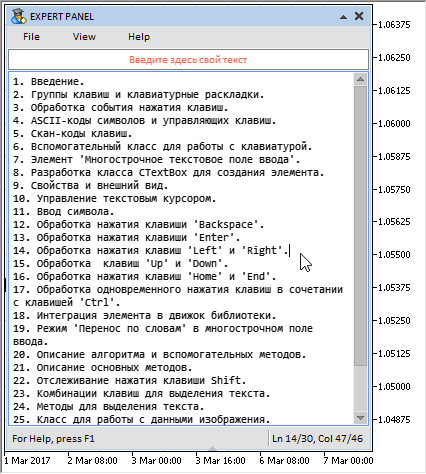### 删除已选文本的方法

```class CTextBox : public CElement
{
private:
//--- 删除一行中选择的文本
void              DeleteTextOnOneLine(void);
};
//+------------------------------------------------------------------+
//| 删除一行中选择的文本                                                 |
//+------------------------------------------------------------------+
void CTextBox::DeleteTextOnOneLine(void)
{
int symbols_total     =::ArraySize(m_lines[m_text_cursor_y_pos].m_symbol);
int symbols_to_delete =::fabs(m_selected_symbol_from-m_selected_symbol_to);
//--- 如果字符的初始索引在右侧
if(m_selected_symbol_to<m_selected_symbol_from)
{
//--- 将字符移动到当前行中的空余区域
MoveSymbols(m_text_cursor_y_pos,m_selected_symbol_from,m_selected_symbol_to);
}
//--- 如果字符的初始索引在左侧
else
{
//--- 将文本光标向左移动要删除数量的字符
m_text_cursor_x_pos-=symbols_to_delete;
//--- 将字符移动到当前行中的空余区域
MoveSymbols(m_text_cursor_y_pos,m_selected_symbol_to,m_selected_symbol_from);
}
//--- 当前行的数组大小减少已提取的字符数
ArraysResize(m_text_cursor_y_pos,symbols_total-symbols_to_delete);
}

```

CTextBox::DeleteTextOnMultipleLines() 方法将用于删除多行选择文本。此处的算法比较复杂。首先, 有必要判断:

• 初始和最后一行的字符总数
• 所选文本的中间行数 (初始行和最后一行除外)
• 初始和最后一行要删除的字符数。

• 从一行中移动到另一行的字符, 即删除后将要保留的内容, 将复制到临时的动态数组当中。
• 调整接收数组 (行) 的大小。
• 数据被添加到接收行结构的数组中。
• 根据删除行的数量移动行。
• 调整行数组大小 (减少已删除的行数)。
• 在初始行高于最后一行 (文本选择向下) 的情况下, 则将 文本光标移动到所选文本的初始索引 (行和字符)
```class CTextBox : public CElement
{
private:
//--- 删除多行中选择的文本
void              DeleteTextOnMultipleLines(void);
};
//+------------------------------------------------------------------+
//| 删除多行中选择的文本                                                 |
//+------------------------------------------------------------------+
void CTextBox::DeleteTextOnMultipleLines(void)
{
//--- 初始和最后一行的字符总数
uint symbols_total_line_from =::ArraySize(m_lines[m_selected_line_from].m_symbol);
uint symbols_total_line_to   =::ArraySize(m_lines[m_selected_line_to].m_symbol);
//--- 要被删除的中间行数
uint lines_to_delete =::fabs(m_selected_line_from-m_selected_line_to);
//--- 初始和最后一行要删除的字符数
uint symbols_to_delete_in_line_from =::fabs(symbols_total_line_from-m_selected_symbol_from);
uint symbols_to_delete_in_line_to   =::fabs(symbols_total_line_to-m_selected_symbol_to);
//--- 如果初始行低于最后一行
if(m_selected_line_from>m_selected_line_to)
{
//--- 将要移动的字符复制到数组中
string array[];
CopyWrapSymbols(m_selected_line_from,m_selected_symbol_from,symbols_to_delete_in_line_from,array);
//--- 调整接收行数组大小
uint new_size=m_selected_symbol_to+symbols_to_delete_in_line_from;
ArraysResize(m_selected_line_to,new_size);
//--- 将数据添加到接收行结构的数组
PasteWrapSymbols(m_selected_line_to,m_selected_symbol_to,array);
//--- 获取行数组的大小
uint lines_total=::ArraySize(m_lines);
//--- 根据要删除的行数向上移动行
MoveLines(m_selected_line_to+1,lines_total-lines_to_delete,lines_to_delete,false);
//--- 调整行数组大小
::ArrayResize(m_lines,lines_total-lines_to_delete);
}
//--- 如果初始行高于最后一行
else
{
//--- 将要移动的字符复制到数组中
string array[];
CopyWrapSymbols(m_selected_line_to,m_selected_symbol_to,symbols_to_delete_in_line_to,array);
//--- 调整接收行数组大小
uint new_size=m_selected_symbol_from+symbols_to_delete_in_line_to;
ArraysResize(m_selected_line_from,new_size);
//--- 将数据添加到接收行结构的数组
PasteWrapSymbols(m_selected_line_from,m_selected_symbol_from,array);
//--- 获取行数组的大小
uint lines_total=::ArraySize(m_lines);
//--- 根据要删除的行数向上移动行
MoveLines(m_selected_line_from+1,lines_total-lines_to_delete,lines_to_delete,false);
//--- 调整行数组大小
::ArrayResize(m_lines,lines_total-lines_to_delete);
//--- 将光标移动到选择的初始位置
SetTextCursor(m_selected_symbol_from,m_selected_line_from);
}
}

```

```class CTextBox : public CElement
{
private:
//--- 删除所选文本
void              DeleteSelectedText(void);
};
//+------------------------------------------------------------------+
//| 删除所选文本                                                       |
//+------------------------------------------------------------------+
bool CTextBox::DeleteSelectedText(void)
{
//--- 如果未选择文本, 离开
if(m_selected_line_from==WRONG_VALUE)
return(false);
//--- 如果从一行中删除字符
if(m_selected_line_from==m_selected_line_to)
DeleteTextOnOneLine();
//--- 如果从多行中删除字符
else
DeleteTextOnMultipleLines();
//--- 重置所选文本
ResetSelectedText();
//--- 计算文本框的大小
CalculateTextBoxSize();
//--- 设置文本框的新尺寸
ChangeTextBoxSize();
//--- 调整滚动条
CorrectingHorizontalScrollThumb();
CorrectingVerticalScrollThumb();
//--- 更新文本框中的文本
DrawTextAndCursor(true);
//--- 发送有关它的消息
::EventChartCustom(m_chart_id,ON_MOVE_TEXT_CURSOR,CElementBase::Id(),CElementBase::Index(),TextCursorInfo());
return(true);
}

```

CTextBox::DeleteSelectedText() 方法不仅在按下 Backspace 键时调用, 而且: (1) 当输入一个新字符并 (2) 按下 Enter 键时调用。在这些情况下, 首先删除文本, 然后执行与按键对应的动作。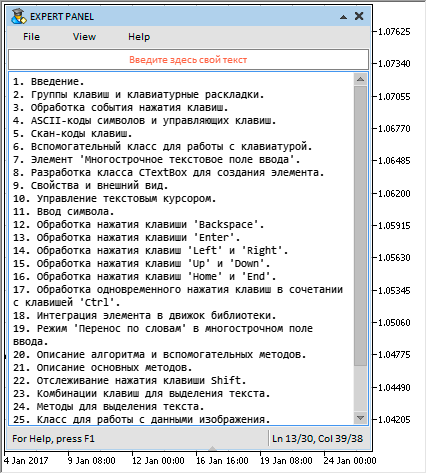### 操纵图像数据的类

• 图像像素数组;
• 图像宽度;
• 图像高度;
• 图像文件路径。
```//+------------------------------------------------------------------+
//| 保存图像数据的类                                                    |
//+------------------------------------------------------------------+
class CImage
{
protected:
uint              m_image_data[]; // 图像像素数组
uint              m_image_width;  // 图像宽度
uint              m_image_height; // 图像高度
string            m_bmp_path;     // 图像文件路径
public:
//--- (1) 数据数组大小, (2) 设置/返回数据 (像素颜色)
uint              DataTotal(void)                              { return(::ArraySize(m_image_data)); }
uint              Data(const uint data_index)                  { return(m_image_data[data_index]);  }
void              Data(const uint data_index,const uint data)  { m_image_data[data_index]=data;     }
//--- 设置/返回图像宽度
void              Width(const uint width)                      { m_image_width=width;               }
uint              Width(void)                                  { return(m_image_width);             }
//--- 设置/返回图像高度
void              Height(const uint height)                    { m_image_height=height;             }
uint              Height(void)                                 { return(m_image_height);            }
//--- 设置/返回图像路径
void              BmpPath(const string bmp_file_path)          { m_bmp_path=bmp_file_path;          }
string            BmpPath(void)                                { return(m_bmp_path);                }

};
//+------------------------------------------------------------------+
//| 构造器                                                            |
//+------------------------------------------------------------------+
CImage::CImage(void) : m_image_width(0),
m_image_height(0),
m_bmp_path("")
{
}
//+------------------------------------------------------------------+
//| 析构器                                                            |
//+------------------------------------------------------------------+
CImage::~CImage(void)
{
}

```

```class CImage
{
public:
//--- 读取并存储所传递的图像数据
};
//+------------------------------------------------------------------+
//| 将所传递的图像保存到一个数组                                          |
//+------------------------------------------------------------------+
{
//--- 清除最后的错误
::ResetLastError();
//--- 保存图像路径
m_bmp_file_path=bmp_file_path;
//--- 读取并保存图像数据
{
::Print(__FUNCTION__," > 错误: ",::GetLastError());
return(false);
}
//---
return(true);
}

```

```class CImage
{
public:
//--- 复制所传递的图像数据
void              CopyImageData(CImage &array_source);
};
//+------------------------------------------------------------------+
//| 复制所传递的图像数据                                                 |
//+------------------------------------------------------------------+
void CImage::CopyImageData(CImage &array_source)
{
//--- 获取接收数组和源数组的大小
uint data_total        =DataTotal();
uint source_data_total =::GetPointer(array_source).DataTotal();
//--- 调整接收数组的大小
::ArrayResize(m_image_data,source_data_total);
//--- 复制数据
for(uint i=0; i<source_data_total; i++)
m_image_data[i]=::GetPointer(array_source).Data(i);
}

```

### 结论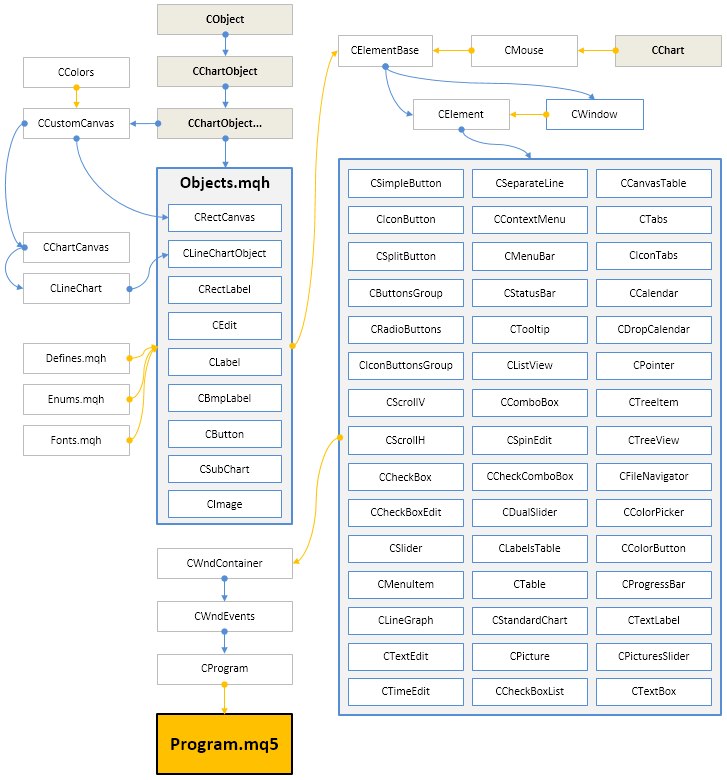## 量化课程

移动端课程